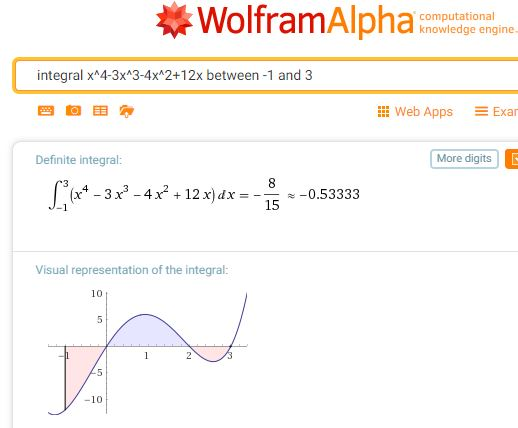# Integration – Desmos & WolframAlpha

With my year 12 students (UK age 16-17) we have been looking at definite Integration. Desmos and WolframAlpha are both excellent for checking work and by using the technology we have a very clear visual representation adding to our understanding.

One of the homework questions for my students involves finding the total shaded area bounded by f(x) = x4−3x3−4x2+12x, the x-axis, the line x=−1 and the line x=3.

We could use WolframAlpha for a quick check. I like the visual representation showing students clearly that they are dealing with areas above and below the x-axis.Scrolling down the page we see that this query also returns the indefinite integral.For the total shaded area, students could change the limits of the query to evaluate each section.

Or we could turn to the excellent Desmos where we can very simply change the limits.

If you are unfamiliar with Integration with Desmos, turn to Learn Desmos: Integrals.
Note you can explore the graph shown in the video.

A version of this post for students which also includes links to some useful notes and examples can be found on Mathematics for Students.